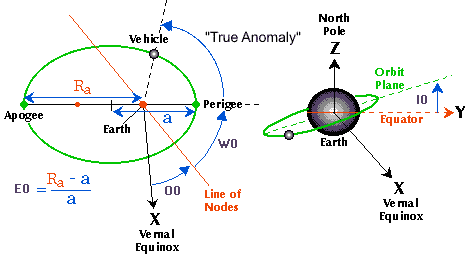## TLEA two-line element set (TLE) is a set of orbital elements that describe the orbit of an earth satellite. A computer program called a model can use the TLE to compute the precise position of a satellite at a particular time. The TLE is in a format specified by NORAD and used by NORAD and NASA. The TLE can be used directly by the SGP4 model (or one of the SGP8, SDP4, SDP8 models). Orbital elements are determined for many thousands of space objects by NORAD and are freely distributed on the Internet in the form of TLEs. A TLE consists of a title line followed by two lines of formatted text. The following is an example of a TLE (for the International Space Station)

ISS (ZARYA)

```ISS (ZARYA)
1 25544U 98067A   04236.56031392  .00020137  00000-0  16538-3 0  5135
2 25544  51.6335 341.7760 0007976 126.2523 325.9359 15.70406856328903

1234567890123456789012345678901234567890123456789012345678901234567890
1         2         3         4         5         6         7```

 Line 0 Columns Example Description 1-24 ISS (ZARYA) the common name for the object based on information from the SatCat. Line 1 Columns Example Description 1 1 Line Number 3-7 25544 Satellite Catalog Number 8 U Elset Classification 10-17 98067A International Designator 19-32 04236.56031392 Element Set Epoch (UTC) 34-43 .00020137 1st Derivative of the Mean Motion with respect to Time 45-52 00000-0 2nd Derivative of the Mean Motion with respect to Time (decimal point assumed) 54-61 16538-3 B* Drag Term 63 0 Element Set Type 65-68 513 Element Number 69 5 Checksum Line 2 Columns Example Description 1 2 Line Number 3-7 25544 Satellite Catalog Number 9-16 51.6335 Orbit Inclination (degrees) 18-25 344.7760 Right Ascension of Ascending Node (degrees) 27-33 0007976 Eccentricity (decimal point assumed) 35-42 126.2523 Argument of Perigee (degrees) 44-51 325.9359 Mean Anomaly (degrees) 53-63 15.70406856 Mean Motion (revolutions/day) 64-68 32890 Revolution Number at Epoch 69 3 Checksum

2-Line Element Definition (meaning table to decode)

```1 AAAAAU YYLLLPPP  BBBBB.BBBBBBBB  .CCCCCCCC  DDDDD-D  EEEEE-E F GGGGZ
2 AAAAA  HHH.HHHH III.IIII JJJJJJJ KKK.KKKK MMM.MMMM NN.NNNNNNNNRRRRRZ```

Meaning

•  – Line #1 label
•  – Line #2 label
• [AAAAA] – Catalog Number assigned sequentially (5-digit integer from 1 to 99999)
• [U] – Security Classification (U = Unclassified)
• [YYLLLPPP] – International Designator (YY = 2-digit Launch Year; LLL = 3-digit Sequential Launch of the Year; PPP = up to 3 letter Sequential Piece ID for that launch)
• [BBBBB.BBBBBBBB] – Epoch Time — 2-digit year, followed by 3-digit sequential day of the year, followed by the time represented as the fractional portion of one day
• [.CCCCCCCC] – ndot/2 Drag Parameter (rev/day2) — one half the first time derivative of the mean motion. This drag term is used by the SGP orbit propagator.
• [DDDDD-D] – n double dot/6 Drag Parameter (rev/day3) — one sixth the second time derivative of the mean motion. The “-D” is the tens exponent (10-D). This drag term is used by the SGP orbit propagator.
• [EEEEE-E] – Bstar Drag Parameter (1/Earth Radii) — Pseudo Ballistic Coefficient. The “-E” is the tens exponent (10-E). This drag term is used by the SGP4 orbit propagator.
• [F] – Ephemeris Type — 1-digit integer (zero value uses SGP or SGP4 as provided in the Project Spacetrack report.
• [GGGG] – Element Set Number assigned sequentially (up to a 4-digit integer from 1 to 9999). This number recycles back to “1” on the update following element set number “9999.”
• [HHH.HHHH] – Orbital Inclination (from 0 to 180 degrees).
• [III.IIII] – Right Ascension of the Ascending Node (from 0 to 360 degrees).
• [JJJJJJJ] – Orbital Eccentricity — there is an implied leading decimal point (between 0.0 and 1.0).
• [KKK.KKKK] – Argument of Perigee (from 0 to 360 degrees).
• [MMM.MMMM] – Mean Anomaly (from 0 to 360 degrees).
• [NN.NNNNNNNN] – Mean Motion (revolutions per day).
• [RRRRR] – Revolution Number (up to a 5-digit integer from 1 to 99999). This number recycles following revolution nymber 99999.
• [Z] – Check Sum (1-digit integer). Both lines have a check sum that is computed from the sum of all integer characters on that line plus a “1” for each negative (-) sign on that line. The check sum is the modulo-10 (or ones digit) of the sum of the digits and negative signs.